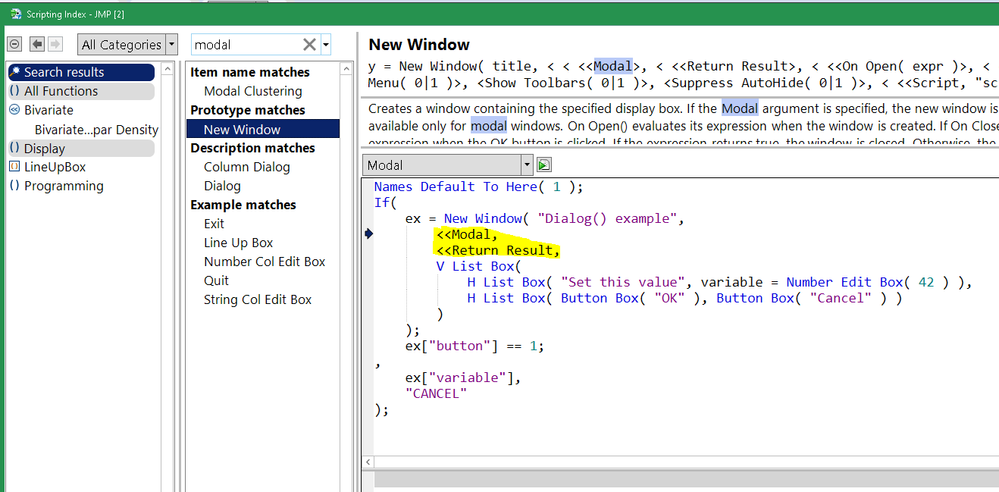Our World Statistics Day conversations have been a great reminder of how much statistics can inform our lives. Do you have an example of how statistics has made a difference in your life? Share your story with the Community!
Choose Language Hide Translation Bar
Highlighted

## Problem when only the file with User Interface

Hi,

The script works ok as a stand alone. it will open and merge the file - no problem.

However, after I build the UI to take more input from user for some calculation (not related to this file open up but involve in some calculation after ), it will show window to ask before it opens each file (but it will run without asking if I run it as a standalone).

I assign the script as "obj" and call out this obj after "ok" button is click.

``````names default to here (1);
dt_raw = current data table();
dt_raw :FW_VERSION << set data type( "character" );

//select parameters for the pareto groupping

charlist = dt_raw << get column names("character");

dt_raw << subset(
all rows,
columns(charlist),
output table("temp")
);

click =0;

nw = new window("Select Parameter for Pareto",
borderbox(left(3),top (2),
hlist box(
textBox("Select Group for Yield Analaysis"),
hlistbox(
vlistbox(
panelbox("Select group",
ColListData = col list box ( data table("temp"),all, << set data type("character"));
)
)
),
panelbox("Select Parameters",
LineupBox(NCol(2),Spacing(3),
ButtonBox("Y, Columns", colListY<<Append(colListData<<GetSelected)),
colListY = ColListBox(nLines(5),"character"),
),

),
panelbox("Action",
button box("OK",
list = eval(colListY<<getitems);
obj;
click =1;
nw << close window;
),
button box("Cancel",nw<<close window),
button box ("Remove",
colListY<<removeselected;
)
)

))
);

//close(data table("Temp"), no save);

// check whether we need to group by Equip ID

if(click ==1, obj);

obj = Expr(

tst = 0;

for(i=1,i<= n items(list),i++,
if(list[i] == "EQUIP_ID",
(remove from(list,i);
tst = 1),
)
);

// Get Spec File
dt_raw  << select where (contains(:Name("~DEVICE TYPE"),"SPC")) << delete rows;
summarize(spec= by(:SPEC_ID));
mypath = "c:\DCO\SPEC\";

i=1;
sname=spec[i] ||".xlsx";
mainDt = Open( mypath || sname);
mainDt << New Column ("source", character, set each value ( spec[i] ));

For( i = 2, i <= n items(spec), i++,
sname=spec[i] ||".xlsx";
dt = Open(mypath || sname ) ;
dt << New Column ("source", character, set each value ( spec[i] ));

mainDt = mainDt << Concatenate( dt, Append to First Table );
Close( dt, NoSave );
Wait( 0.1 );
);

mainDt<< Set Name( "Spec Files" );
data table("Spec Files") << delete columns(:LongDescriptor,:Result,:Units,:Regrade,:Status,:Format,:Store,:Table);
data table("Spec Files") << select where(ismissing(:Minimum)&ismissing(:Maximum));
data table("Spec Files") << delete rows;

);``````
1 ACCEPTED SOLUTION

Accepted Solutions
Highlighted

## Re: Problem when only the file with User Interface

I think you want to make the dialog modal, so it blocks execution until it is dismissed.modal dialog in scripting index

Craigemodal dialog in scripting index